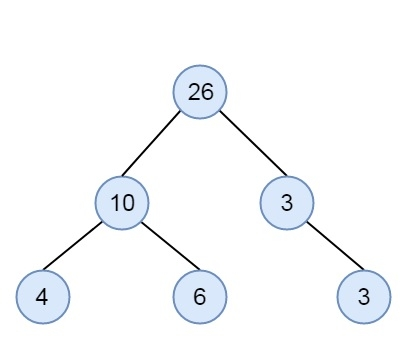# Check if a given Binary Tree is SumTree in C++

Here we will see how to check whether a binary tree is sum-tree or not. Now the question is what is the sum-tree. A sum-tree is a binary tree where a node will hold the sum value of its children. The root of the tree will contain an entire sum of all elements below it. This is an example of sum-tree −To check this, we will follow a simple trick, we will find the sum of left and right subtree elements if the sum value is the same as the root, then that is sum-tree. This will be one recursive approach.

## Example

Live Demo

#include <bits/stdc++.h>
using namespace std;
class node {
public:
int data;
node* left, *right;
};
int sum_of_nodes(node *root) {
if(root == NULL)
return 0;
return sum_of_nodes(root->left) + root->data + sum_of_nodes(root->right);
}
int isSumTree(node* node) {
int left_sum, right_sum;
if(node == NULL || (node->left == NULL && node->right == NULL))
return 1;
left_sum = sum_of_nodes(node->left);
right_sum = sum_of_nodes(node->right);
if((node->data == left_sum + right_sum) && isSumTree(node->left) && isSumTree(node->right))
return 1;
return 0;
}
node* getNode(int data) {
node* newNode = new node();
newNode->data = data;
newNode->left = newNode->right = NULL;
return newNode;
}
int main() {
node *root = getNode(26);
root->left = getNode(10);
root->right = getNode(3);
root->left->left = getNode(4);
root->left->right = getNode(6);
root->right->right = getNode(3);
if(isSumTree(root))
cout << "The tree is Sum Tree";
else
cout << "The tree is not a Sum Tree";
}

## Output

The tree is Sum Tree